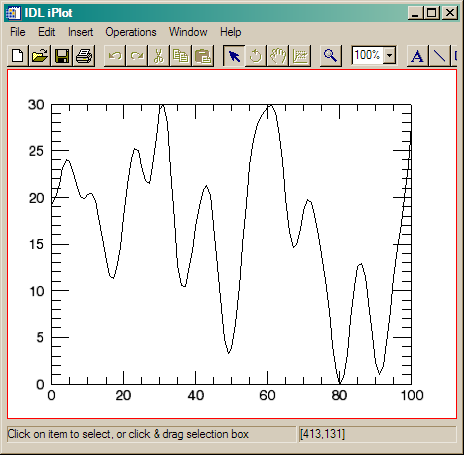Filling the Plot Window

QUESTION: Is there any way to programmatically make the plot in an iPlot window actually occupy more than just a small portion of the graphics window? Perhaps I'm thick, but this has been bugging me for a while, and I can't figure out how to do it.ANSWER: The answer to this question comes from Mike Galloy in a 6 April 2007 article on the IDL Newsgroup.

Yes, I would appreciate an easy way to do this too, like an XMARGIN and YMARGIN keywords that work like the PLOT commands. Currently, I get the visualization layer and set the VIEWPLANE_RECT property to get the margins I want.

There will be (presumably) a FIT_TO_VIEW keyword in IDL 6.4 which will make the visualization to take up the entire view.

In the meantime, here is a wrapper for iPlot that handles margins for the plot.

;+
; Wrapper routine for iPlot which handles margins for the plot also.
;
; @param x {in}{required}{type=1D numeric array}
;        x-coordinates of data if y param is passed or y-coordinates of data
;        if only x is passed
; @param y {in}{optional}{type=1D numeric array}
;        y-coordinates of data
; @keyword xmargin {in}{optional}{type=fltarr(2)}{default=[0.1, 0.1]}
;          size of left and right margins in window normal units
; @keyword ymargin {in}{optional}{type=fltarr(2)}{default=[0.1, 0.1]}
;          size of bottom and top margins in window normal units
; @keyword _extra {in}{optional}{type=keywords}
;          keywords to iPlot
;-
pro iplot_with_margins, x, y, xmargin=xmargin, ymargin=ymargin, _extra=e
compile_opt strictarr

myXMargin = n_elements(xmargin) eq 0 ? [0.1, 0.1] : xmargin
myYMargin = n_elements(ymargin) eq 0 ? [0.1, 0.1] : ymargin

case n_params() of
0 : iplot, _strict_extra=e
1 : iplot, x, _strict_extra=e
2 : iplot, x, y, _strict_extra=e
endcase
toolID = itGetCurrent(tool=oTool)

visIds = oTool->findIdentifiers('*', /visualization)
visLayerId = strmid(visIds, 0, strpos(visIds, '/', / reverse_search))
oVisLayer = oTool->getByIdentifier(visLayerId)

xsize = 1.4 / (1.0 - myXMargin - myXMargin)
ysize = 0.98 / (1.0 - myYMargin - myYMargin)
xstart = - myXMargin * xsize - 1.4 / 2
ystart = - myYMargin * ysize - 0.98 / 2

oVisLayer->setProperty, viewplane_rect=[xstart, ystart, xsize, ysize]
endUsing the wrapper program by Mike Galloy, the initial plot in an iPlot window fills the available space in the graphics window.Web Coyote's Guide to IDL Programming